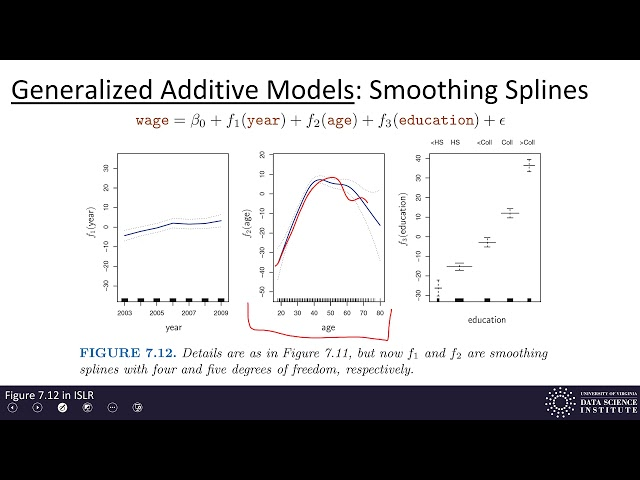# What is Generalized additive models

##### Introduction

Generalized Additive Models (GAMs) are a flexible and powerful framework for regression analysis, which can be applied to a wide range of problems in statistics, machine learning, and data science. GAMs extend linear regression models by allowing non-linear relationships between the dependent variable and multiple predictor variables. This makes them especially useful for modeling complex, non-linear relationships in data without making any assumptions about the underlying distribution of the data.

##### What is a GAM?

GAM is a generalized linear model in which the linear predictor depends on smooth functions of predictors. In other words, GAM involves systematic function generation from data-driven curves that allow for both linear and non-linear associations among predictors and outcome variables. GAMs are used to fit many types of data because the function curves can be different from linear predictors, thus expressing the model with sufficient flexibility.

##### How does GAM work?

Like traditional linear regression models, GAMs predict a continuous dependent variable (often referred to as the response variable) based on a set of independent variables (often referred to as predictors). However, unlike linear regression models, GAMs allow for non-linear relationships between the predictors and the dependent variable by using non-parametric smoothing functions.

Non-parametric smoothing functions are functions that do not have a predetermined functional form, but are instead estimated from the data. Typically, these functions are fitted using techniques like splines or kernel smoothing. The specific smoothing function used depends on the nature of the data and the research question being addressed.

• GAMs can model complex, non-linear relationships between the dependent variable and predictor variables without requiring any assumptions about the underlying distribution of the data.
• GAMs can overcome issues with collinearity and complex interactions between predictor variables, which can be difficult to account for in traditional linear regression models.
• GAMs are highly flexible and can be adapted to suit a wide range of applications across various domains.
• GAMs can model relationships using different types of smoothing functions, such as splines or kernel smoothing, depending on the research question.
• GAMs can be used to handle missing data by including the missing data as another predictor variable.

• GAMs can be computationally intensive and can require a large amount of data in order to produce reliable results.
• GAMs are sensitive to the choice of smoothing function used and can produce different results depending on the choice of smoothing function.
• GAMs can be difficult to interpret, especially when multiple smoothing functions are used.
• GAMs can be susceptible to overfitting if the number of predictor variables and/or the complexity of the smoothing functions used is too high.
##### Applications of GAMs

GAMs are useful in a wide range of applications across various domains, including:

• Ecology: modeling the relationship between species abundance and environmental variables such as temperature and rainfall.
• Economics: modeling the relationship between economic variables such as GDP, inflation, and unemployment.
• Healthcare: modeling the relationship between patient outcomes and predictor variables such as age, gender, and comorbidities.
• Meteorology: modeling the relationship between weather variables such as temperature, rainfall, and wind speed.
##### Conclusion

Generalized Additive Models (GAMs) are a powerful and flexible framework for modeling relationships between predictor variables and a dependent variable. GAMs enable modeling of nonlinear relationships between variables and provide a more data-driven approach to capturing relationships than traditional linear regression models. GAMs have numerous applications in a variety of fields, including ecology, economics, healthcare, and meteorology.